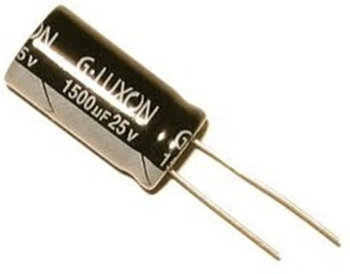﻿ How Much Does a Capacitor Charge To After One Time Constant?

# How Much Does a Capacitor Charge To After One Time Constant?A capacitor charges to 63% of the supply voltage that is charging it after one time period.

After one time period, which is calculated by the formula, τ=RC, a capacitor will be charged to 63% of the supply voltage.

### Example

Below we have a circuit of a 9-volt battery charging a 1000µF capacitor through a 3KΩ resistor:One time constant, τ=RC=(3KΩ)(1000µF)=3 seconds. So after 3 seconds, the capacitor is charged to 63% of the 9 volts that the battery is supplying it, which would be approximately 5.67 volts.